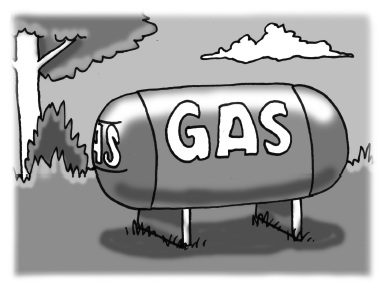### Home > CCA2 > Chapter 2 > Lesson 2.2.1 > Problem2-94

2-94.People who live in isolated or rural areas often have their own tanks that hold propane gas to run appliances like stoves, washers, and water heaters. Some of these tanks are made in the shape of a cylinder with two hemispheres on the ends, as shown in the picture at right. (Recall that a hemisphere is half of a sphere, and the volume of a sphere is found by using $V=\frac{4}{3}\pi r^3$.)

The Inland Propane Gas Tank Company wants to make tanks with this shape, and to offer models in different sizes. The cylindrical portion of each of the different tanks will be $4$ meters long. However, the radius, $r$, will vary among the different models.

1. One of the tank models has a radius of $1$ meter. What is its volume?

Substitute $1$ for $r$ in the sphere and cylinder equations.

$V=4\pi$

$\textit{V}=\frac{4}{3}\pi$

$\textit{V}=4\pi + \frac{4}{3}\pi$

$\frac{16}{3}\pi \approx 16.755 \: \text{m}^3$

2. If the radius is doubled, will the volume double? Explain. Then calculate the volume of the larger tank with $r=2$ m.

Remember that the radius is being cubed.

The radius will not double because of the $r:r^2:r^3$ relationship.

Now, see part (a).

$\textit{V}=16\pi + \frac{32}{3}\pi$

The tank will be
$83.776m^3$.

3. Write an equation that will let the Inland Propane Gas Tank Company determine the volume of a tank with any size radius.

Remember that the total is the combination of a sphere and a cylinder.

$\textit{V}=\frac{4}{3}\pi\textit{r}^{3}+4\pi\textit{r}^{2}$